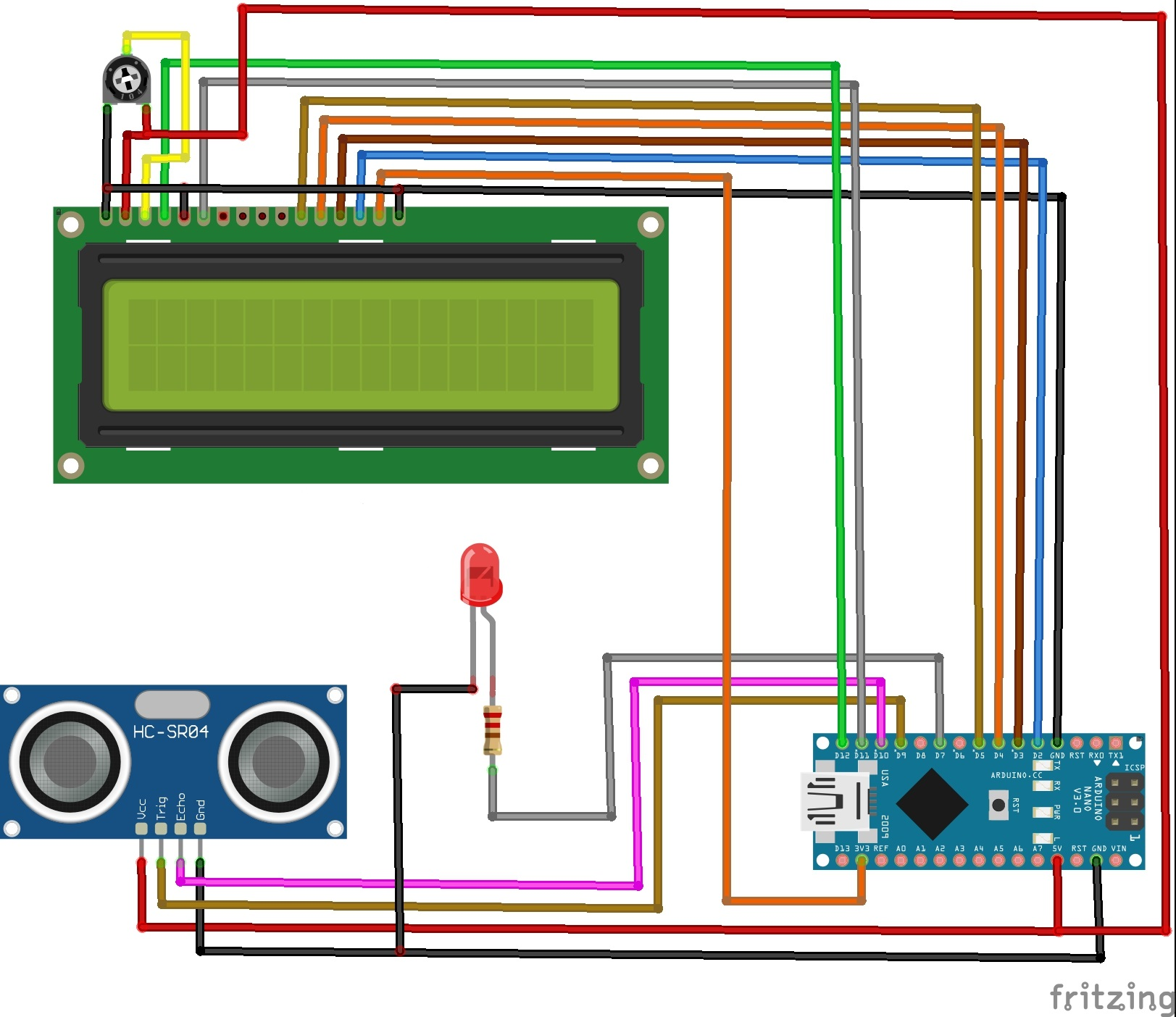Hello,

In this tutorial, we are going to make a speedometer using Arduino.

## Circuit Diagram

####• The anode pin is connected to 3.3V on the Arduino and the cathode pin is connected to the GND pin.
• The four data pins of the LCD are connected to the digital pins 2 to 5 of the Arduino board.
• The Vo pin is connected to the power supply of the LCD via a potentiometer to control the contrast and brightness of the LCD.
• Register pin (RS) is connected to the digital pin 12 or Arduino.
• The R/W pin is to be set to low as LCD is the output device here. So we will connect this pin to the GND on the Arduino.
• The enable pin is used to enable the LCD. This pin is connected to the digital pin 11 on the Arduino board.
• The VCC and GND pins of the Sensor are connected to 5V and GND respectively.
• The TRIG pin is connected to digital pin 9.
• The ECHO pin is connected to digital pin 10.

## Working concept

• The working concept of this project is very simple and consists of three main components: the LCD, ultrasonic sensor and the Arduino.
• The unit used in this project for speed is cm/s.
• The ultrasonic sensor takes reading every second. Let us consider the distance at first second distance1 and the distance after that to be distance 2.
• So the speed can be calculated by subtracting the distances after each second.

Speed = |distance1 - distance2|

• We used the modulus sign so that the speed stays positive when the object is coming close or going away.

## Arduino Code

• Upload the code to the Arduino board.
• You can use whichever case you want. Make it out of cardboard or any other material. You can even make your own design and 3D print the case.